﻿ 2023年信息与数学学院学术报告（五）-长江大学信息与数学学院首页学术信息正文

### 2023年信息与数学学院学术报告（五）

models with subcritical-critical nonlinearities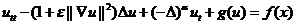,

where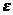[0, 1] is a perturbed extensibility parameter,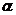[1/2, 1) is a dissipative index. We show that when the nonlinearity g(u) is of either critical growth as(1/2, 1) or subcritical growth as= 1/2, while h(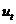) is of critical growth depending the, the model is well-posed and its weak solution is exactly the strong one; the related solution semigroup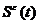has a strong (X, Y )-global attractor and a strong(X, Y )-exponential attractor, which are also the standard global and exponential attractor of optimal regularity ofin X, respectively, where X is the energy space and Y is the strong solution space; these global attractors are upper semicontinuous and these exponential attractors are Holder continuous with respect to perturbed parameterin the sense of Y -topology, respectively. This work is joint with Fang Da and Yue Sun.